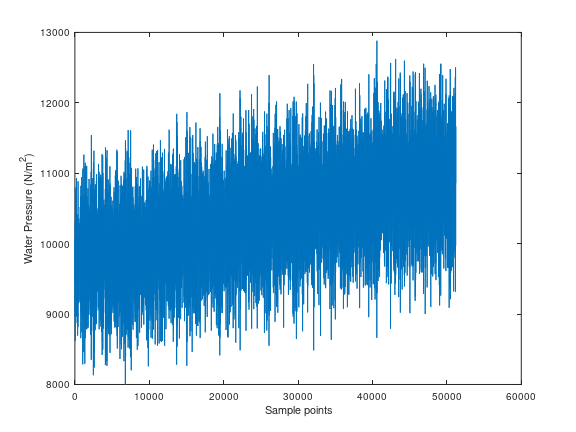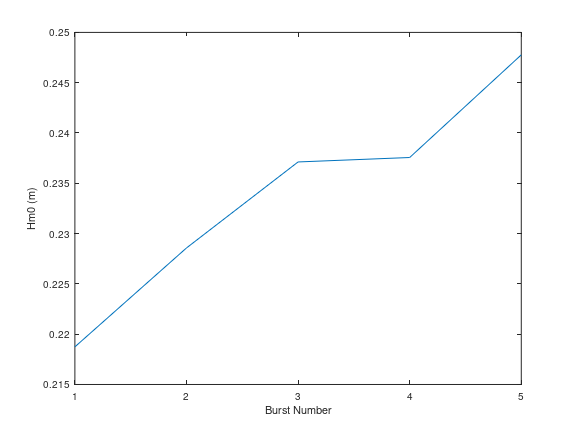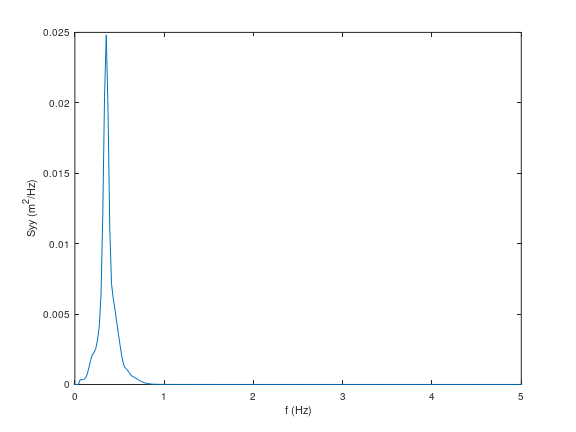# Example (MATLAB Version)¶

Here is an example shows how to use OCEANLYZ. In this example, we use a provided sample file “waterpressure_5burst.csv” as input data. This sample file contains five bursts of water pressure data recorded with a pressure sensor. Sample file may be downloaded at https://github.com/akarimp/oceanlyz/releases/tag/2.0 .

Measurement properties for “waterpressure_5burst.csv” are:

Properties Value OCEANLYZ Properties
File name waterpressure_5burst.csv
Data type Water pressure obj.InputType=’pressure’
Number of recorded burst (n_burst) 5 obj.n_burst=5
Sampling frequency (fs) 10 (Hz) obj.fs=10
Recording duration (burst_duration) 1024 (second) obj.burst_duration=1024
Pressure sensor height from bed (heightfrombed) 0.05 (m) obj.heightfrombed=0.05
Mean water depth (h) Varies in each burst

To start using OCEANLYZ, first, we need to be in a folder that contains OCEANLYZ files. Assume OCEANLYZ files are in ‘C:\oceanlyz_matlab’. First, we change current working directory to OCEANLYZ folder as:

cd('C:\oceanlyz_matlab') %Change current working directory to OCEANLYZ folder


Next, we download water pressure dataset (“waterpressure_5burst.csv”), we unzip it and copy sample files in a desired folder.

Assume we are currently in OCEANLYZ folder ‘C:\oceanlyz_matlab’ and downloaded sample data file is stored in ‘C:\oceanlyz_matlab\Sample_Data’. Then, we load data as:

current_folder = pwd;                %Current (OCEANLYZ) path
cd('C:\oceanlyz_matlab\Sample_Data') %Change current folder to a folder that contains data file
cd(current_folder)                   %Change current folder back to initial (OCEANLYZ) folder


We can plot data if we need to as:

plot(water_pressure)
xlabel('Sample points')
ylabel('Water Pressure (N/m^2)')Figure 1: Plot of input water pressure data

Then, we need to create an OCEANLYZ object as:

%Create OCEANLYZ object
ocn = oceanlyz;


Next, we assign wave data to OCEANLYZ object as:

%Input data
ocn.data = water_pressure;


Now, we set up OCEANLYZ properties as:

ocn.InputType='pressure';
ocn.OutputType='wave+waterlevel';
ocn.AnalysisMethod='spectral';
ocn.n_burst=5;
ocn.burst_duration=1024;
ocn.fs=10;
ocn.fmin=0.05;
ocn.fmax=ocn.fs/2;
ocn.fmaxpcorrCalcMethod='auto';   %Only required if ocn.InputType='pressure'
ocn.Kpafterfmaxpcorr='constant';  %Only required if ocn.InputType='pressure'
ocn.fminpcorr=0.15;               %Only required if ocn.InputType='pressure'
ocn.fmaxpcorr=0.55;               %Only required if ocn.InputType='pressure'
ocn.heightfrombed=0.05;           %Only required if ocn.InputType='pressure'
ocn.dispout='yes';
ocn.Rho=1024;                     %Seawater density (Varies)


After all required properties are set, we can run OCEANLYZ as:

ocn.runoceanlyz()


Output is stored as a structure array. Name of output is ‘oceanlyz_object.wave’. Field(s) in this structure array can be called by using ‘.’ For example oceanlyz_object.wave.Hm0 contains zero-moment wave height and oceanlyz_object.wave.Tp contains peak wave period.

Here we show how to plot zero-moment wave height:

Hm0 = ocn.wave.Hm0; %zero-moment wave height
plot(Hm0)
xlabel('Burst Number')
ylabel('Hm0 (m)')Figure 2: Plot of $$H_{m0}$$ versus burst number

Similarly, we can plot wave spectrum for the first burst:

f = ocn.wave.f; %frequency of the first burst
Syy = ocn.wave.Syy; %spectrum of the first burst
plot(f(1,:),Syy(1,:))
xlabel('f (Hz)')
ylabel('Syy (m^2/Hz)')Figure 3: Plot of $$S_{yy}$$ versus f